Methods and formulas for tests of fixed effects in Fit Mixed Effects Model

Select the method or formula of your choice.

Tests of fixed effect terms

The tests of the fixed effect terms are F tests. The null hypothesis for the test depends on whether the test is for a fixed factor term or a covariate term. For a fixed factor term, the null hypothesis is that the term does not significantly affect the response. For a covariate term, the null hypothesis is that no association exists between the response and the covariate term.

Minitab offers 2 methods to test fixed effect terms: Kenward-Roger approximation and Satterthwaite approximation. For more information on Kenward-Roger approximation, see Kenward and Roger.1 For more information on Satterthwaite approximation, see Giesbrecht and Burns 2 as well as Fai and Cornelius. 3

The calculation of the denominator degrees of freedom for the F-statistic and the calculation of the F-statistic differ. The calculation of the numerator degrees of freedom and the determination of a p-value for a given F-statistic are the same for both methods.

Kenward-Roger approximation

Kenward-Roger approximation is one method to test the statistical significance of fixed effect terms.

where

Notation

TermDescription
lthe numerator degrees of freedom, which is the number of parameters in the term to test
0the matrix with 0 components
Ilthe identity matrix with l dimension
c + 1the number of variance components
wrs(r, s)th component of the asymptotic variance-covariance matrix of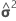V−1the inverse of the variance-covariance matrix

For further details on the notation, go to the Methods section.

where

Kenward and Roger λ

The value of the Kenward and Roger λ depends on two conditions.
••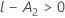If both conditions are true, then the formula follows:

If either condition is not true, then λ = 1.

Under the null hypothesis, lambda × F is asymptotically F distributed with degrees of freedom DF Num, and DF Den. The calculation of the P-value uses this property.

Satterthwaite approximation

Satterthwaite approximation is one method to test the statistical significance of fixed effect terms.

F-statistic

where L and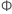have the same definitions as in Kenward-Roger approximation.

Denominator degrees of freedom

The process for the determination of the degrees of freedom has multiple steps.

1. Perform the spectral decomposition on the variance of the fixed effect parameter vector estimate:

where P is an orthogonal matrix of eigenvectors and D is a diagonal matrix of eigenvalues, both of dimension l × l.

2. Define lr to be the rth row of P'L, r = 1, ..., l and let

where dr is the rth diagonal element of D, W is the asymptotic variance covariance matrix of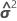and gr is the gradient vector of the following elements:

where

i = 1, …, c, and

3. Let

where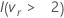is an indicator function that eliminates terms with4. The denominator degrees of freedom depend on the value of E.

• If E > l then the degrees of freedom follow:
• Otherwise, DF Den = 1

Numerator degrees of freedom (DF Num)

The degrees of freedom for a fixed effect depend on the type of effect.
Effect DF
Fixed Factor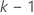Covariate 1
Interactions that involve fixed factors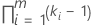Notation

TermDescription
kthe number of levels in the fixed factor term
mthe number of factors in the interaction

P-value – Tests of fixed effects

The p-value is calculated by the following expression:

Notation

TermDescription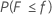the cumulative distribution function of the F-distribution with degrees of freedom equal to DF Num and DF Den, respectivelythe calculated F-value for a term
1 Kenward, M.G. and Roger, J. H. (1997). Small Sample Inference for Fixed Effects from Restricted Maximum Likelihood. Biometrics, Vol 53, No. 3 pp 983-997 .
2 Giesbrecht, F.G. and Burns, J. C. (1985). Two-Stage Analysis Based on a Mixed Model: Large-Sample Approximation Theory and Small-Sample Simulation Results Biometrics, Vol. 41, No. 2 pp 477 - 486.
3 Fai, A. H. and Cornelius, P. L. (1996) Approximate F-Tests of Multiple Degree of Freedom Hypotheses in Generalized Least Squares Analyses of Unbalanced Split-Plot Experiments J. Statist. Comput. Simul. Vol. 54, pp 363-378
By using this site you agree to the use of cookies for analytics and personalized content.  Read our policy